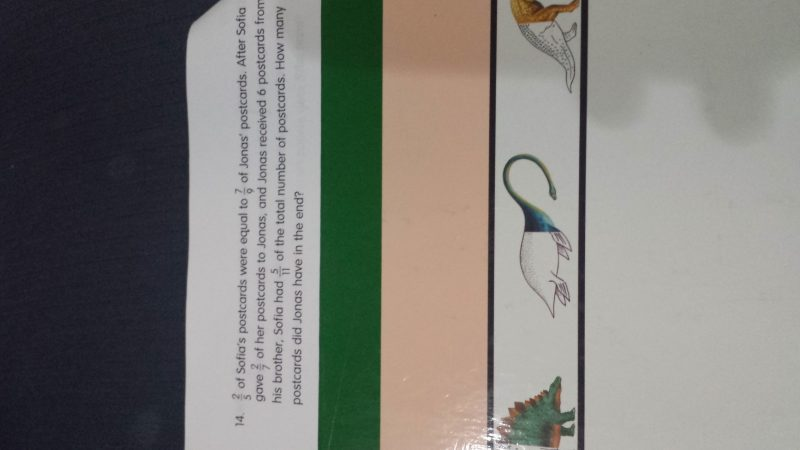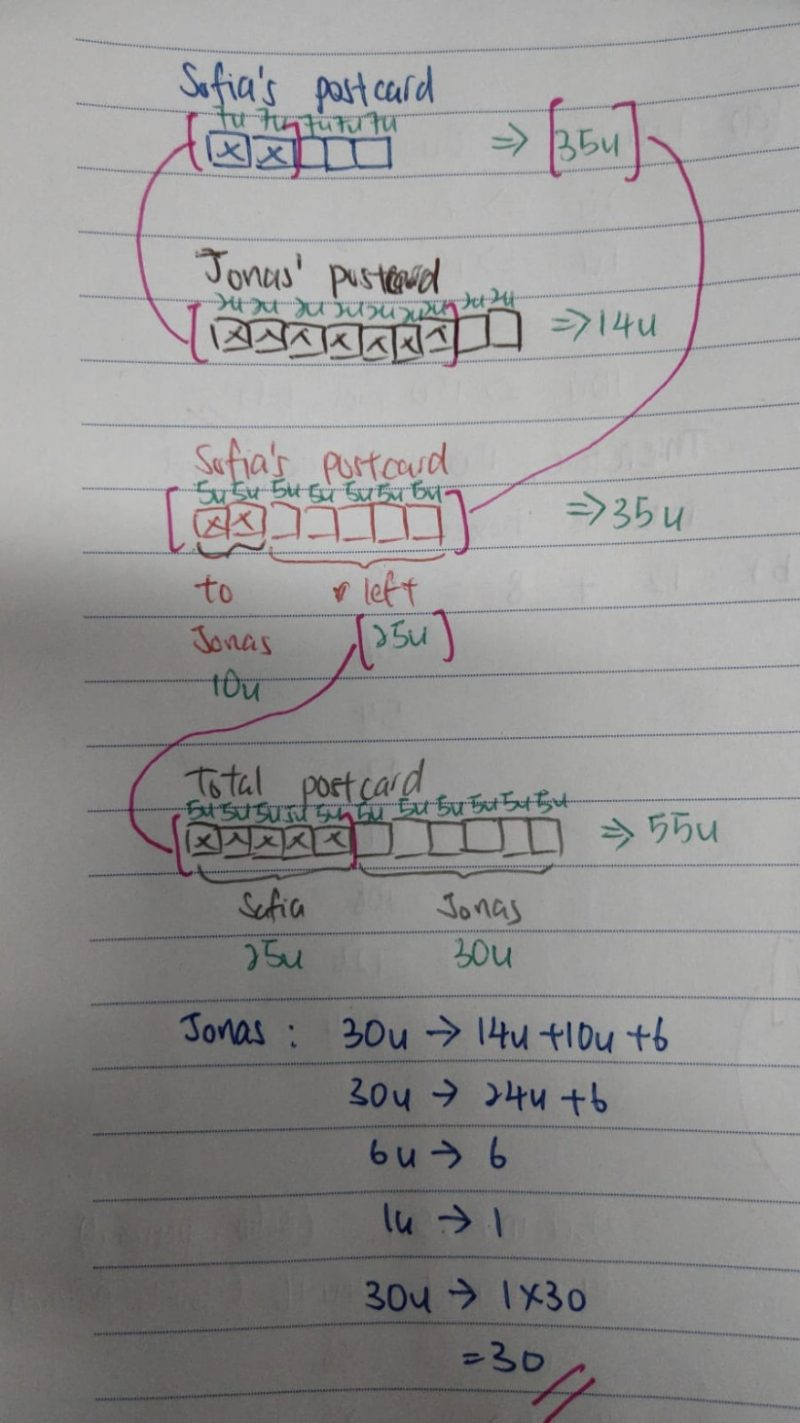# QuestionYou are most welcome.

0 Replies 0 Likes

Method 1 :
Sofia : 7 units + 7 units + 7 units + 7 units + 7 units = 35 units
Jonas : 7 units + 7 units + 4 units = 18 units
(2/7) x 35 = 10
35 units – 10 units = 25 units
10 units + 6 + 18 units = 28 units + 6
11 – 5 = 6
25 units/(28 units + 6) = 5/6
150 units = 140 units + 30
1 unit = 3
28 units + 6 = 28 x 3 + 6 = 90

Method 2 :
2/5 = 14/35
Sofia : 35 units
7/9 = 14/18
Jonas : 18 units
(2/7) x 35 = 10
35 – 10 = 25
18 + 10 = 28
25 units/(28 units + 6) = 5/6
150 units = 140 units + 30
1 unit = 3
28 units + 6 = 28 x 3 + 6 = 90

Ans : 90 postcards.

0 Replies 0 Likes